If a shape is divided into four equal parts it has been quartered.

# Fractions of Shapes

This Math quiz is called 'Fractions of Shapes' and it has been written by teachers to help you if you are studying the subject at elementary school. Playing educational quizzes is an enjoyable way to learn if you are in the 3rd, 4th or 5th grade - aged 8 to 11.

It costs only \$12.50 per month to play this quiz and over 3,500 others that help you with your school work. You can subscribe on the page at Join Us

Finding fractions of shapes means being able to understand that a shape split into two equal parts has been halved, that a shape split into four equal parts (perhaps by halving and then halving again) has been quartered and a shape split into three equal parts is in thirds. Children should recognize the equivalence of two quarters equaling one half, and also that three quarters is greater than a half, but less than the whole. Using division is key when working out and finding fractions of whole numbers.

Use this quiz to practice finding fractions of shapes.

Question 1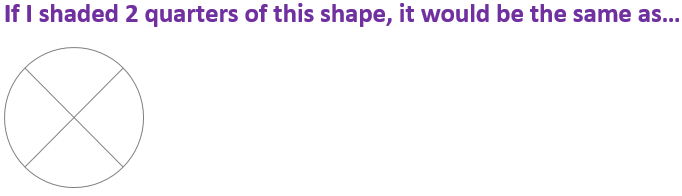12
34
25
14
There are four quarters. If I shaded two of them, it would be half of the shape
Question 2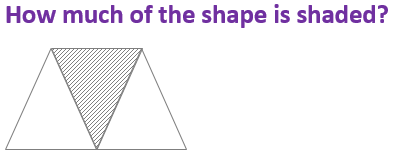23
14
13
12
One of the three equal parts is called a third
Question 3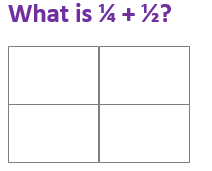24
14
34
12
If you shaded in 1 quarter, and then 2 more, you would have shaded 3 of the 4 quarters
Question 4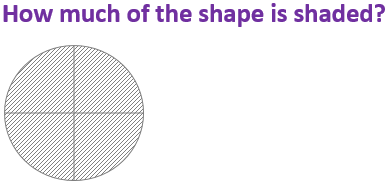24
14
44
12
The whole shape is shaded – this is 4 quarters
Question 5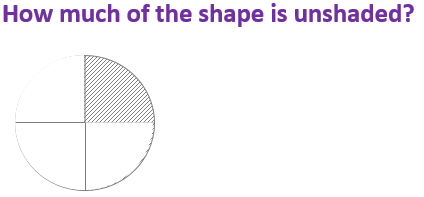24
34
44
14
3 of the 4 quarters are not shaded, only one quarter is shaded
Question 6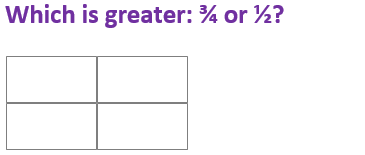24
They are both the same
12
34
12 is the same as 24 so 34 is greater
Question 7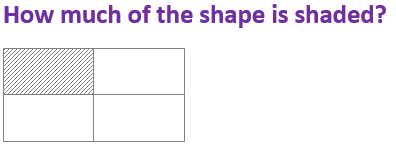24
14
12
34
The shape is divided into four equal sections – each one is a quarter. One is shaded
Question 8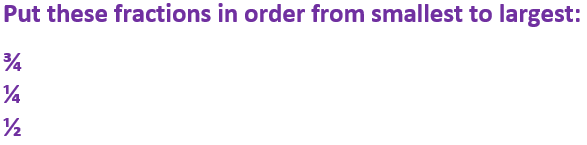34, 14, 12
14, 34, 12
12, 14, 34
14, 12, 34
12 is the same as 24
Question 9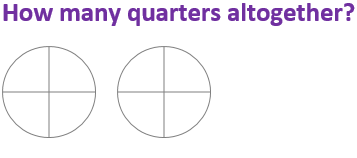8
6
5
10
Each shape has 4 quarters so there are 8 altogether
Question 10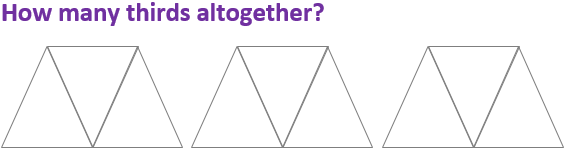5
3
6
9
Each shape has 3 thirds. There are 3 shapes so 3 x 3 = 9
Author:  Angela Smith